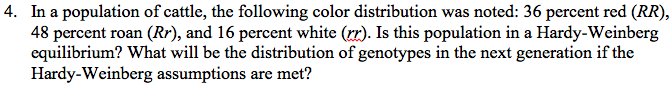# Problem: In a population of cattle, the following color distribution was noted: 36 percent red (RR), 48 percent roan (Rr), and 16 percent white (rr). Is this population in a Hardy-Weinberg equilibrium? What will be the distribution of genotypes in the next generation if the Hardy-Weinberg assumptions are met?

###### FREE Expert Solution

For problems involving Hardy-Weinberg equilibrium, the relative frequency of the dominant allele is usually represented by p, and for the recessive allele, it is represented by q. Moreover, the genotypic frequencies can be expressed as p2 for homozygous dominant, q2 for the homozygous recessive, and 2pq for heterozygous.###### Problem Details

In a population of cattle, the following color distribution was noted: 36 percent red (RR), 48 percent roan (Rr), and 16 percent white (rr). Is this population in a Hardy-Weinberg equilibrium? What will be the distribution of genotypes in the next generation if the Hardy-Weinberg assumptions are met?